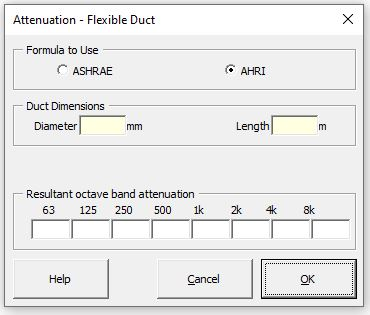### Strutt Help

Attenuation - Flexible Duct    1/1

Strutt|Mechanical Services|Duct Elements|Flexible inserts octave band adjustments into the active row of the worksheet.The values for the ASHRAE method for flexible duct attenuation are sourced from Table 25 (ASHRAE 2019).

The values for the AHRI method are calculated using the empirical formula from Table D9 (AHRI 885-2008):

IL = C1 + C2 \cdot D + C3 \ cdot D^2 + (C4 + C5 \cdot D + C6 \cdot D^2) L

where,
IL is the insertion loss for each octave band
L is the length of the duct, in feet (the upper bound of L is 3 m (10 ft))
D is the diameter of the flex duct, in inches
C1...C6 are empirical coefficients for each octave band centre frequency, given in Table D9 of AHRI 885-2008.

It is noted that the empirical formula appears to overestimate the insertion loss at 125 Hz compared to the tabulated data in Table D9.

Both methods are applicable for flexible ducts for a diameter up to 400 mm (16 in). The input required is the diameter (mm or in) and the length (m or ft).

Both methods interpolate the insertion loss for lengths below the lower bound of the empirical data (i.e. for lengths below 0.9 m); use the interpolated insertion losses with caution.

References:

• ASHRAE 2019 Handbook p.47.25
• AHRI 885-2008, Table D9